Courses

# Optimal Power System Operation of Power Systems

## 10 Questions MCQ Test Topicwise Question Bank for Electrical Engineering | Optimal Power System Operation of Power Systems

Description
This mock test of Optimal Power System Operation of Power Systems for Electrical Engineering (EE) helps you for every Electrical Engineering (EE) entrance exam. This contains 10 Multiple Choice Questions for Electrical Engineering (EE) Optimal Power System Operation of Power Systems (mcq) to study with solutions a complete question bank. The solved questions answers in this Optimal Power System Operation of Power Systems quiz give you a good mix of easy questions and tough questions. Electrical Engineering (EE) students definitely take this Optimal Power System Operation of Power Systems exercise for a better result in the exam. You can find other Optimal Power System Operation of Power Systems extra questions, long questions & short questions for Electrical Engineering (EE) on EduRev as well by searching above.
QUESTION: 1

### If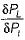for a plant i with output P1 and loss in the system being PL increases, the penalty factor of the system

Solution:

Penalty factor of the system is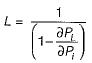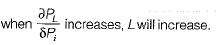QUESTION: 2

### The incremental fuel costs of two plants are given by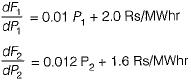For economic schedule of a load of 180 MW

Solution:

For economic scheduling of plants, we have: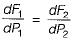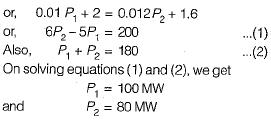QUESTION: 3

### A generator is supplying a load. An incremental change in load of 4 MW requires generation to be increased by 6 MW. The cost at plant bus is ₹ 30/MWhr. The incremental cost at the receiving end is

Solution: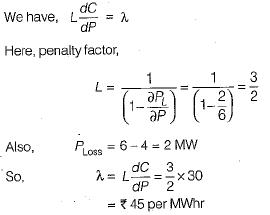QUESTION: 4

The current distribution factors for the power system network shown below are given by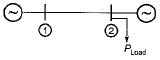Solution:

Since the load is at plant 2 therefore,
Na2 = 0 and Na1 = 1

QUESTION: 5

The incremental transmission loss of a plant can’t be a

Solution:

The incremental transmission loss of a plant can be positive, a non-zero value but, can’t be zero.

QUESTION: 6

When generating units are loaded to equal incremental costs, it results in

Solution:

To minimize the total fuel cost, the generating units must operate at equal incremental cost of production.

QUESTION: 7

Unit of heat rate curve is

Solution: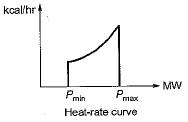QUESTION: 8

Unit of λ is

Solution: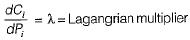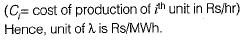QUESTION: 9

Cost curves are expressed as:

Solution:
QUESTION: 10

The optimization problem is

Solution: